1. Nested for loops for printing¶

A simple example of two nested for loops to generate the multiplication table.

In :
for i in range(2, 6): # This is called the "outer loop"
for j in range(2, 6): # This is called the "inner loop"
print(i, 'x', j, '=', i*j)
2 x 2 = 4
2 x 3 = 6
2 x 4 = 8
2 x 5 = 10
3 x 2 = 6
3 x 3 = 9
3 x 4 = 12
3 x 5 = 15
4 x 2 = 8
4 x 3 = 12
4 x 4 = 16
4 x 5 = 20
5 x 2 = 10
5 x 3 = 15
5 x 4 = 20
5 x 5 = 25

What happens if we add conditionals in the outer and/or inner loop? Predict the output of the following examples:

In :
for i in range(2, 6):
for j in range(2, 6):
if i <= j:
print(i, 'x', j, '=', i*j)
2 x 2 = 4
2 x 3 = 6
2 x 4 = 8
2 x 5 = 10
3 x 3 = 9
3 x 4 = 12
3 x 5 = 15
4 x 4 = 16
4 x 5 = 20
5 x 5 = 25
In :
for i in range(2, 6):
if i % 2 == 0:
for j in range(2, 6):
if i <= j:
print(i, 'x', j, '=', i*j)
2 x 2 = 4
2 x 3 = 6
2 x 4 = 8
2 x 5 = 10
4 x 4 = 16
4 x 5 = 20

As another simple example of nested loops, let's see one that prints words. Predict the words that will be printed, in order.

In :
for letter in ['b','d','r','s']:
for suffix in ['ad', 'ib', 'ump']:
print(letter + suffix)
bib
bump
dib
dump
rib
rump
sib
sump

2. Exercise 1: Multiplication Tables¶

Write a multiplication table of dimension yRows * xCols where yRows represents the number of rows in the table and xCols represents the number of columns in the table.

multTable(4, 4)

1 2 3 4

2 4 6 8

3 6 9 12

4 8 12 16

multTable(3, 5)

1 2 3 4 5

2 4 6 8 10

3 6 9 12 15

Hint: In Python, you can change print() to end with something other than the newline character (i.e., '\n') by changing its default argument. To do so, simply write print('a', end = ''). The parameter end sets how you should conclude printing. By default, it is '\n'. Now it is '', allowing the programmer to continue printing on the same line.

Hint: Remember also that in Python you can print one line at a time moving from left to right. This means you can't print each full column as you go. This will be important when you decide what role the inner for loop and outer for loop should play.

In :

def multTable(yRows, xCols):
"""A simple function to print a multiplication table."""
for y in range(1, yRows + 1):
for x in range(1, xCols + 1):
print(x * y, end = ' ')
print('\n')

In :
multTable(4, 4)
1 2 3 4

2 4 6 8

3 6 9 12

4 8 12 16

In :
multTable(3, 5)
1 2 3 4 5

2 4 6 8 10

3 6 9 12 15

You may notice that when you call multTable with arguments larger than 4 or 5 that the table starts to become noticeably misaligned.

In :
multTable(9, 9)
1 2 3 4 5 6 7 8 9

2 4 6 8 10 12 14 16 18

3 6 9 12 15 18 21 24 27

4 8 12 16 20 24 28 32 36

5 10 15 20 25 30 35 40 45

6 12 18 24 30 36 42 48 54

7 14 21 28 35 42 49 56 63

8 16 24 32 40 48 56 64 72

9 18 27 36 45 54 63 72 81

In lab, you will learn how to align the columns so that your multiplication table is more aesthetically pleasing.

3. Nested loops for accumulation¶

A common reason to use nested loops is to accumulate values for every element of a sequence:

In :
def isVowel(char):
return char.lower() in 'aeiou'

verse = "Two roads diverged in a yellow wood"
for word in verse.split():
counter = 0
for letter in word:
if isVowel(letter):
counter += 1
print('Vowels in "' + word + '" =>', counter)
Vowels in "Two" => 1
Vowels in "diverged" => 3
Vowels in "in" => 1
Vowels in "a" => 1
Vowels in "yellow" => 2
Vowels in "wood" => 2

Experiment!¶

In the code above change the position of the statement counter = 0.

1. take it outside the outer loop
2. put it inside the inner loop

how can you explain these results?

Experiment 1: take counter outside the outer loop

In :
def isVowel(char):
return char.lower() in 'aeiou'

verse = "Two roads diverged in a yellow wood"
counter = 0

for word in verse.split():
for letter in word:
if isVowel(letter):
counter += 1
print('Vowels in "' + word + '" =>', counter)
Vowels in "Two" => 1
Vowels in "diverged" => 6
Vowels in "in" => 7
Vowels in "a" => 8
Vowels in "yellow" => 10
Vowels in "wood" => 12

Explanation: Here counter is accumulating the vowels across all words in the sentence, thus, at the end it contains the total number of vowels.

Experiment 2: put counter inside the inner loop

In :
def isVowel(char):
return char.lower() in 'aeiou'

verse = "Two roads diverged in a yellow wood"

for word in verse.split():
for letter in word:
counter = 0           # counter inside the inner loop
if isVowel(letter):
counter += 1
print('Vowels in "' + word + '" =>', counter)
Vowels in "Two" => 1
Vowels in "diverged" => 0
Vowels in "in" => 0
Vowels in "a" => 1
Vowels in "yellow" => 0
Vowels in "wood" => 0

Explanation: Here counter is set to 0 every time we enter the inner loop and it forgets about vowels that it has seen before.

4. Avoiding nested loops with functions¶

Since nested loops can be challenging to think about, it's nice to try to avoid them when possible.

One strategy is to capture the inner loop in a separate function. In the above vowel-counting example, this is easy to do with the countVowels function we defined in a previous lecture:

In :
def isVowel(char):
"""Return true if char is a vowel."""
return char.lower() in 'aeiou'

def countVowels(word):
"""Return the number of vowels in a provided word."""
counter = 0
for letter in word:
if isVowel(letter):
counter += 1
return counter

def countVowelsInVerse(verse):
"""Return the number of vowels in a provided phrase."""
for word in verse.split():
print('Vowels in "' + word + '" =>', countVowels(word))

countVowelsInVerse("Two roads diverged in a yellow wood")
Vowels in "Two" => 1
Vowels in "diverged" => 3
Vowels in "in" => 1
Vowels in "a" => 1
Vowels in "yellow" => 2
Vowels in "wood" => 2

By "hiding" the inner loop in the countVowels function, we end up thinking about two independent non-nested loops!

5. Exercise 2: Nested loops with graphics¶

In this exercise, we will create grids of circles like those shown in the pictures below.

 Circle Grid with Light Sky Blue Circle Grid with Random Colors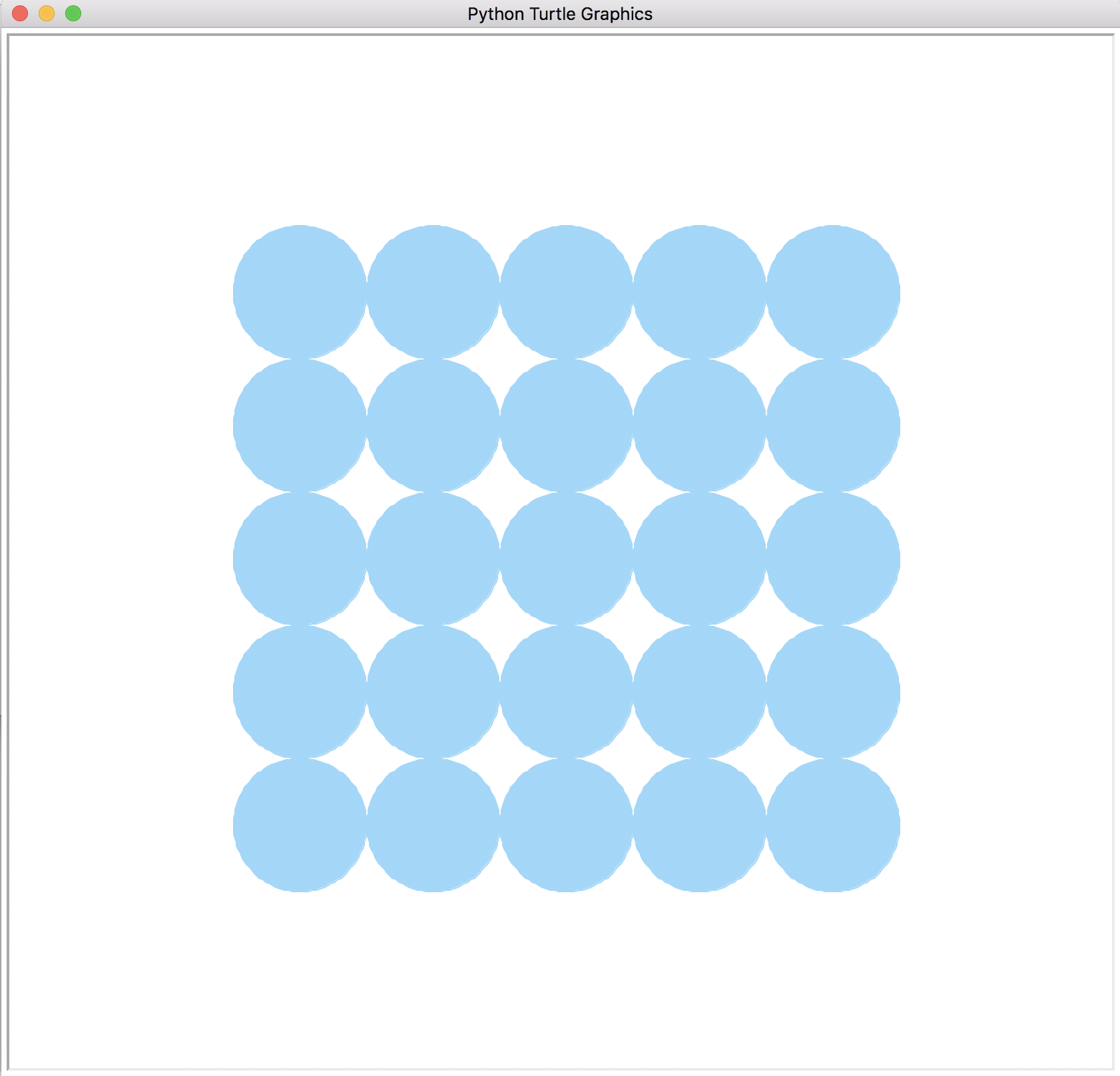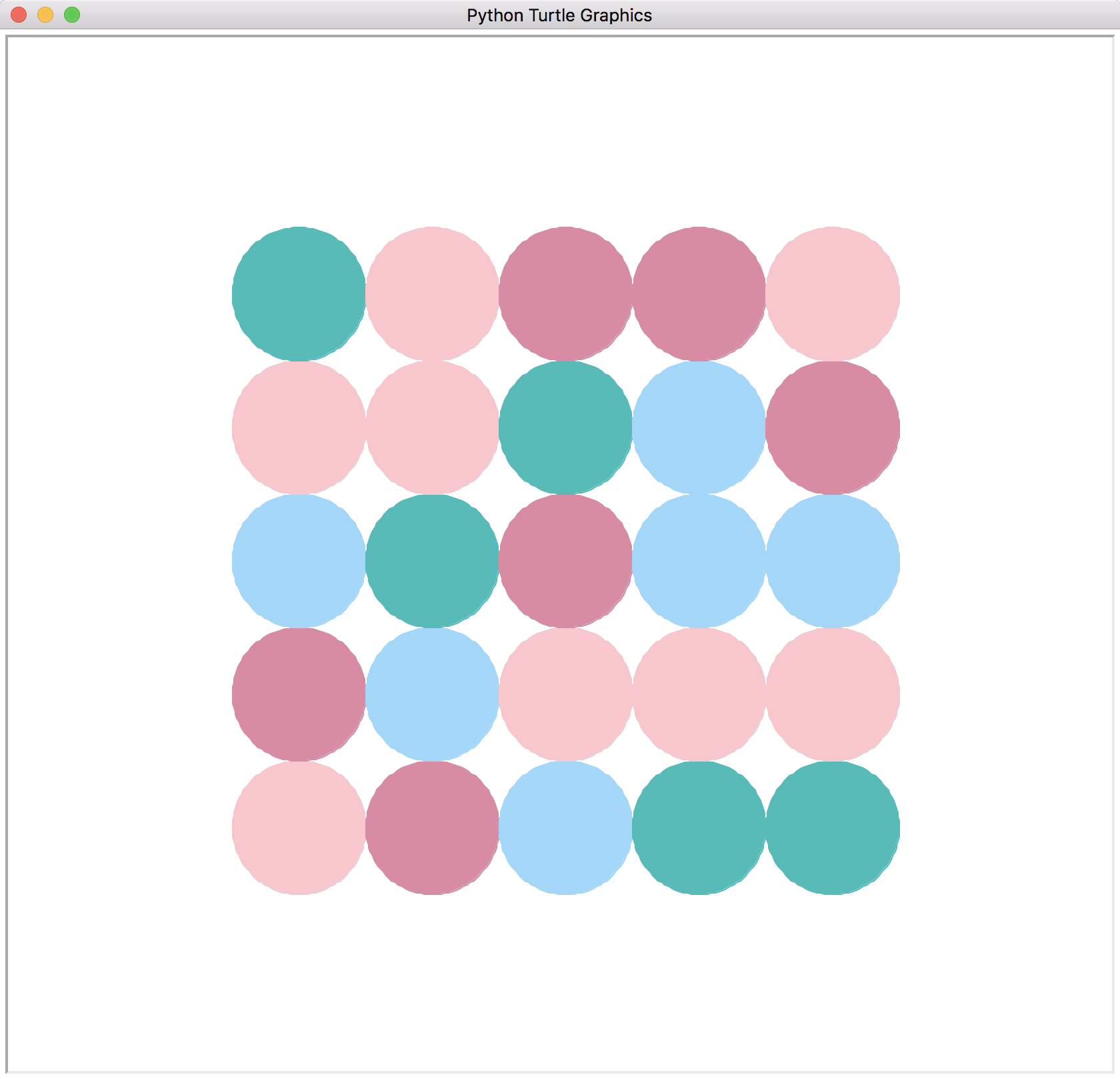First lets start out by importing the necessary turtle functions into Python.

In :
from turtle import *

Before we start drawing things, it would be nice to write a function that can figure out the coordinates for any point in the grid. That way, when we are writing our loops, we can think about rows and columns, but not have to worry about exact Turtle coordinates. Here's a function that takes row and column indices along with the number of desired rows, columns, and circle radius, and returns a pair of coordinates for the indicated position:

In :
def gridCoordinates(row, col, numRows, numCols, radius):
offsetX = (numCols * cellSize) / 2 - radius
offsetY = (numRows * cellSize) / 2 - radius
return (cellSize*col - offsetX, cellSize*row - offsetY) # note that column -> x and row -> y

Note: the way this function is defined, each row will be higher than the last, with row 0 at the bottom, and each column will be farther right than the last, with column 0 at the left.

Next we will write a nested loop that prints out the grid coordinates (rows and columns).

Step 1: Print a sequence of row and column numbers¶

Define a function printGridIndices that takes two arguments: a width and a height. It should print out all of the coordinate pairs within the given width/height grid, starting at 0, 0, and ending at width-1, height-1.

Reminder: You can use range to iterate through a sequence of numbers.

Example output:

printGridIndices(3, 3)
0 0
0 1
0 2
1 0
1 1
1 2
2 0
2 1
2 2
In :
def printGridIndices(width, height):
for row in range(height):
for col in range(width):
print(row, col)
In :
printGridIndices(3, 3)
0 0
0 1
0 2
1 0
1 1
1 2
2 0
2 1
2 2

Step 2: Draw circles with nested loops¶

We've got a loop that prints out the row and column numbers from our grid, and the gridCoordinates function from above can convert these into turtle x, y positions. Now all we have to do is put those things together with calls to teleport and drawCircle to draw a grid of circles. Write the blueCircleGrid function below, using LightSkyBlue as the color for each circle.

Note: Because gridCoordinates returns a pair, it can be convenient to use tuple assignment to receive that pair, like this:

x, y = gridCoordinates(row, col, radius)

This code takes the pair returned by the gridCoordinates function and assigns the first item to the variable x and the second item to the variable y.

In :
for row in range(numRows):
for col in range(numCols):
x, y = gridCoordinates(row, col, numRows, numCols, radius)
color("LightSkyBlue")
teleport(x, y)
begin_fill()
end_fill()
In :
# Test for the blueCircleGrid function:
reset()
noTrace()
blueCircleGrid(4, 7, 50)
showPicture()
A 1-pensize LightSkyBlue circle centered at (-300, -150) with radius 50
A 1-pensize LightSkyBlue circle centered at (-200, -150) with radius 50
A 1-pensize LightSkyBlue circle centered at (-100, -150) with radius 50
A 1-pensize LightSkyBlue circle centered at (0, -150) with radius 50
A 1-pensize LightSkyBlue circle centered at (100, -150) with radius 50
A 1-pensize LightSkyBlue circle centered at (200, -150) with radius 50
A 1-pensize LightSkyBlue circle centered at (300, -150) with radius 50
A 1-pensize LightSkyBlue circle centered at (-300, -50) with radius 50
A 1-pensize LightSkyBlue circle centered at (-200, -50) with radius 50
A 1-pensize LightSkyBlue circle centered at (-100, -50) with radius 50
A 1-pensize LightSkyBlue circle centered at (0, -50) with radius 50
A 1-pensize LightSkyBlue circle centered at (100, -50) with radius 50
A 1-pensize LightSkyBlue circle centered at (200, -50) with radius 50
A 1-pensize LightSkyBlue circle centered at (300, -50) with radius 50
A 1-pensize LightSkyBlue circle centered at (-300, 50) with radius 50
A 1-pensize LightSkyBlue circle centered at (-200, 50) with radius 50
A 1-pensize LightSkyBlue circle centered at (-100, 50) with radius 50
A 1-pensize LightSkyBlue circle centered at (0, 50) with radius 50
A 1-pensize LightSkyBlue circle centered at (100, 50) with radius 50
A 1-pensize LightSkyBlue circle centered at (200, 50) with radius 50
A 1-pensize LightSkyBlue circle centered at (300, 50) with radius 50
A 1-pensize LightSkyBlue circle centered at (-300, 150) with radius 50
A 1-pensize LightSkyBlue circle centered at (-200, 150) with radius 50
A 1-pensize LightSkyBlue circle centered at (-100, 150) with radius 50
A 1-pensize LightSkyBlue circle centered at (0, 150) with radius 50
A 1-pensize LightSkyBlue circle centered at (100, 150) with radius 50
A 1-pensize LightSkyBlue circle centered at (200, 150) with radius 50
A 1-pensize LightSkyBlue circle centered at (300, 150) with radius 50

Step 3: Draw the circles with random colors¶

Now make your grid randomly select the color of each circle from a list of colors. To perform this task, first import random.

In :
import random

To randomly select from a list of colors, we can use the function random.choice(sequence) where sequence is any sequence like a list, range, or string. Notice how running the cells below may produce a different result each time.

In :
random.choice([1, True, -4.5, "Hello"])
Out:
-4.5
In :
random.choice(range(100, 200, 15))
Out:
145
In :
random.choice("strings are sequences of letters")
Out:
'f'

Now let's define a list of colors below:

In :
colors = ["LightSkyBlue", "LightPink", "LightSeaGreen", "PaleVioletRed"]

Below, define the randomCircleGrid function to create a grid of circles with a randomly chosen color from the list above.

In :
for row in range(numRows):
for col in range(numCols):
x, y = gridCoordinates(row, col, numRows, numCols, radius)
color(random.choice(colors))
teleport(x, y)
begin_fill()
end_fill()
In :
# Test for randomCircleGrid:
reset()
noTrace()
randomCircleGrid(5, 6, 50)
showPicture()
A 1-pensize LightSkyBlue circle centered at (-250, -200) with radius 50
A 1-pensize LightSeaGreen circle centered at (-150, -200) with radius 50
A 1-pensize PaleVioletRed circle centered at (-50, -200) with radius 50
A 1-pensize PaleVioletRed circle centered at (50, -200) with radius 50
A 1-pensize PaleVioletRed circle centered at (150, -200) with radius 50
A 1-pensize PaleVioletRed circle centered at (250, -200) with radius 50
A 1-pensize LightPink circle centered at (-250, -100) with radius 50
A 1-pensize LightSeaGreen circle centered at (-150, -100) with radius 50
A 1-pensize PaleVioletRed circle centered at (-50, -100) with radius 50
A 1-pensize PaleVioletRed circle centered at (50, -100) with radius 50
A 1-pensize LightSeaGreen circle centered at (150, -100) with radius 50
A 1-pensize LightSeaGreen circle centered at (250, -100) with radius 50
A 1-pensize LightSkyBlue circle centered at (-250, 0) with radius 50
A 1-pensize PaleVioletRed circle centered at (-150, 0) with radius 50
A 1-pensize LightSkyBlue circle centered at (-50, 0) with radius 50
A 1-pensize LightSkyBlue circle centered at (50, 0) with radius 50
A 1-pensize LightSkyBlue circle centered at (150, 0) with radius 50
A 1-pensize LightSeaGreen circle centered at (250, 0) with radius 50
A 1-pensize LightSkyBlue circle centered at (-250, 100) with radius 50
A 1-pensize PaleVioletRed circle centered at (-150, 100) with radius 50
A 1-pensize LightSkyBlue circle centered at (-50, 100) with radius 50
A 1-pensize PaleVioletRed circle centered at (50, 100) with radius 50
A 1-pensize PaleVioletRed circle centered at (150, 100) with radius 50
A 1-pensize LightSkyBlue circle centered at (250, 100) with radius 50
A 1-pensize PaleVioletRed circle centered at (-250, 200) with radius 50
A 1-pensize LightPink circle centered at (-150, 200) with radius 50
A 1-pensize LightPink circle centered at (-50, 200) with radius 50
A 1-pensize LightPink circle centered at (50, 200) with radius 50
A 1-pensize LightSkyBlue circle centered at (150, 200) with radius 50
A 1-pensize LightPink circle centered at (250, 200) with radius 50

6. Exercise 3: Triangles of Circles¶

Suppose we want to generate triangular patterns of circles that touch two sides of a square:

 UpperLeftTriangleOfCircles UpperRightTriangleOfCircles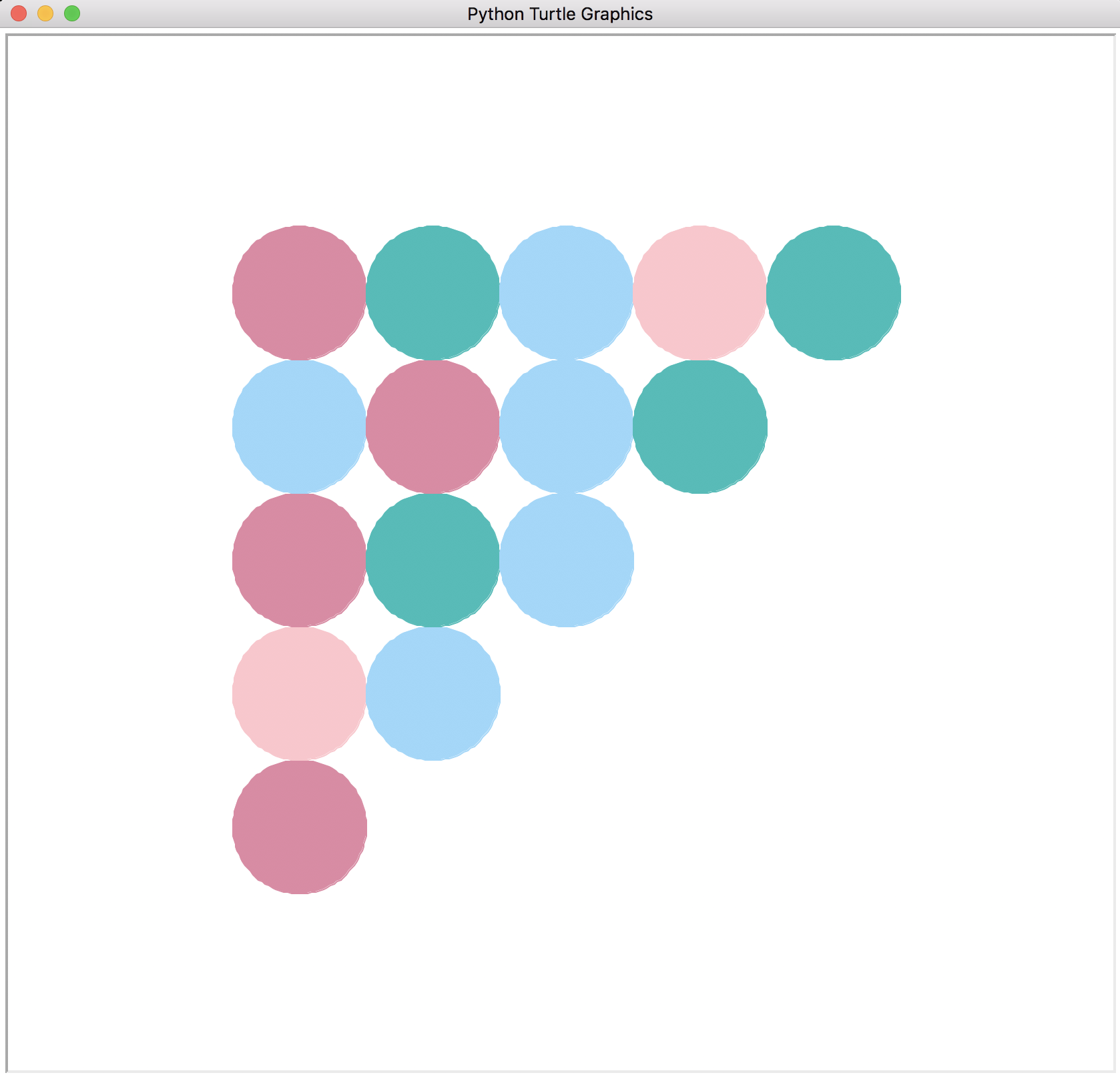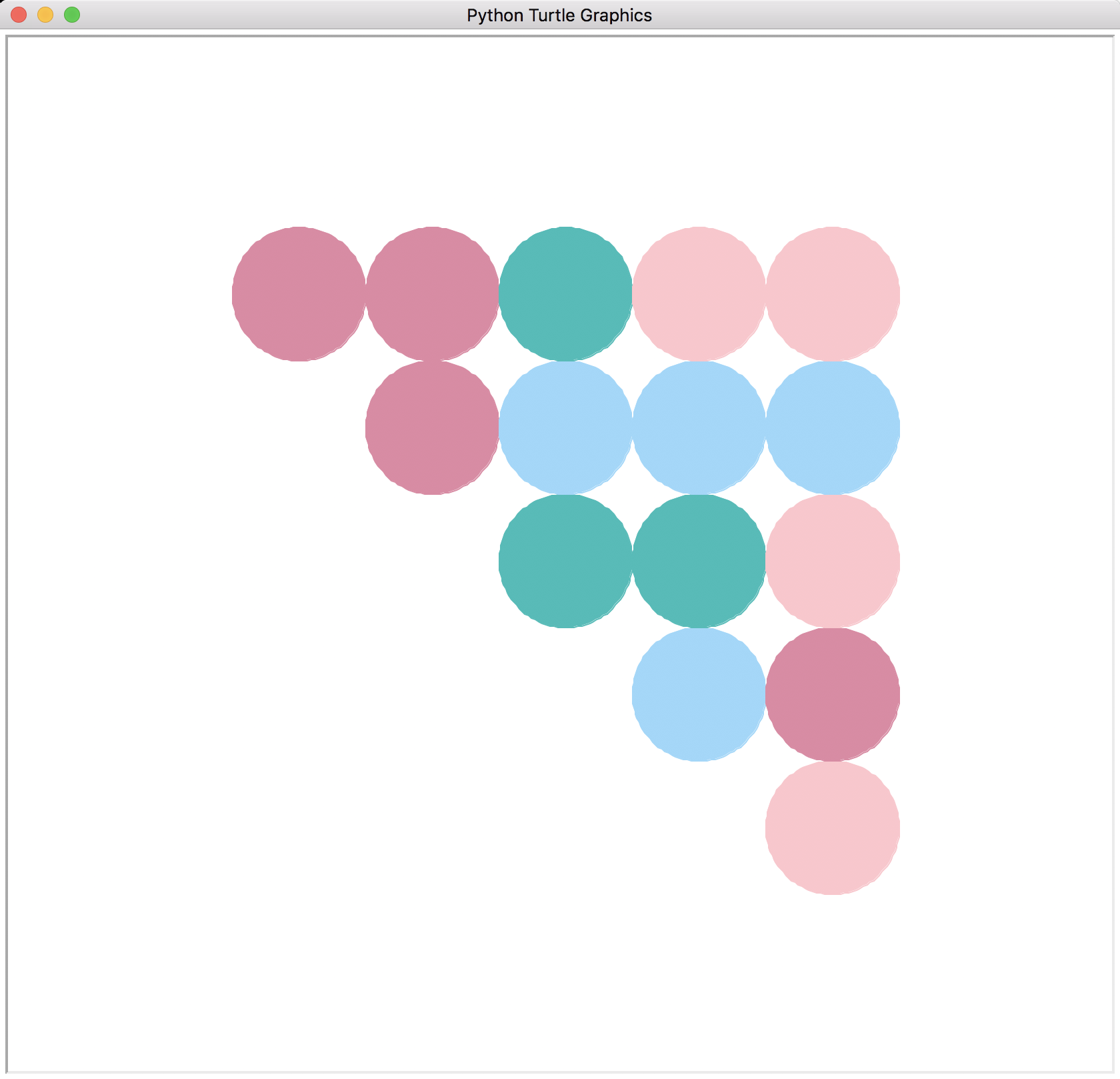LowerLeftTriangleOfCircles LowerRightTriangleOfCircles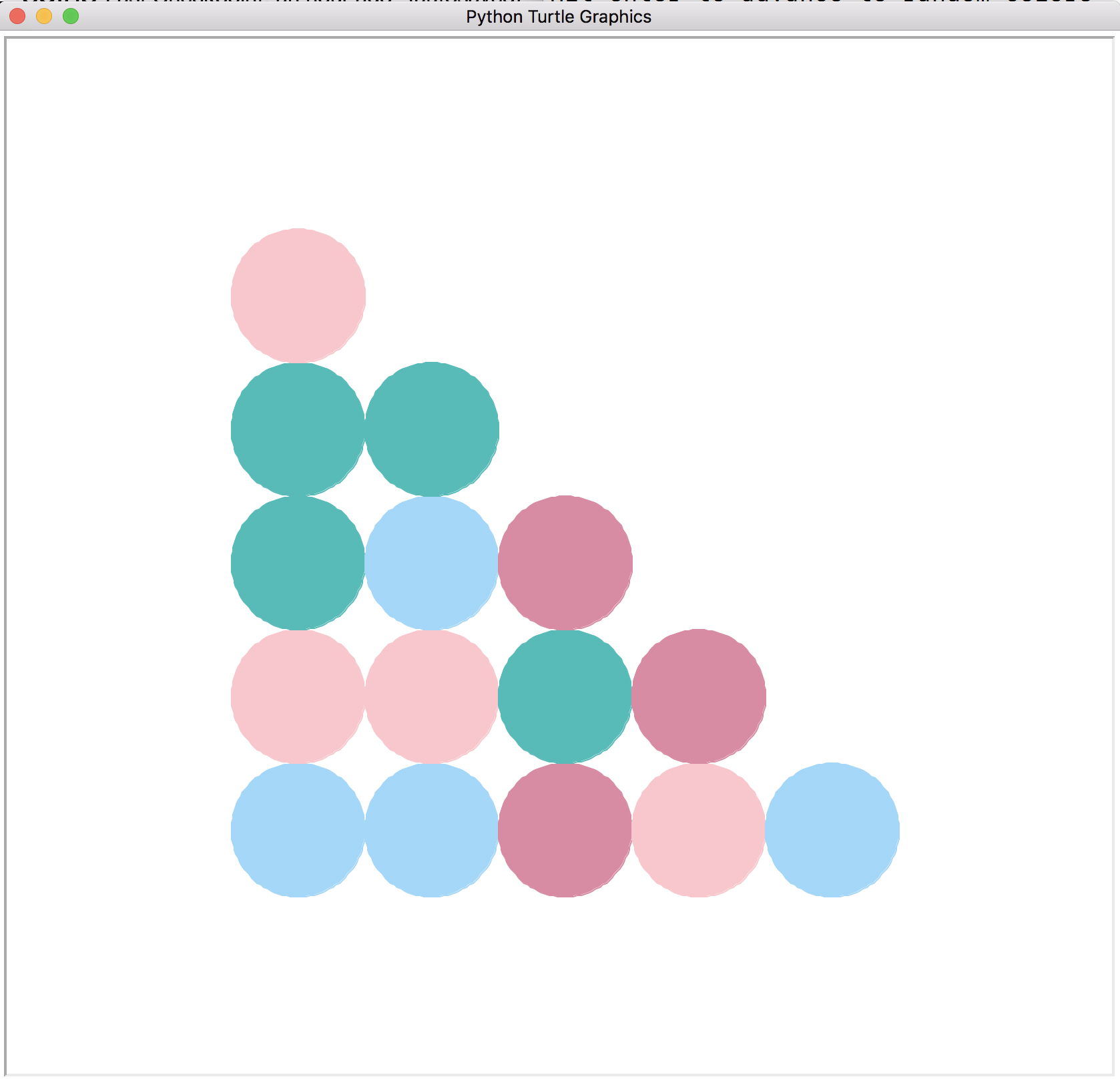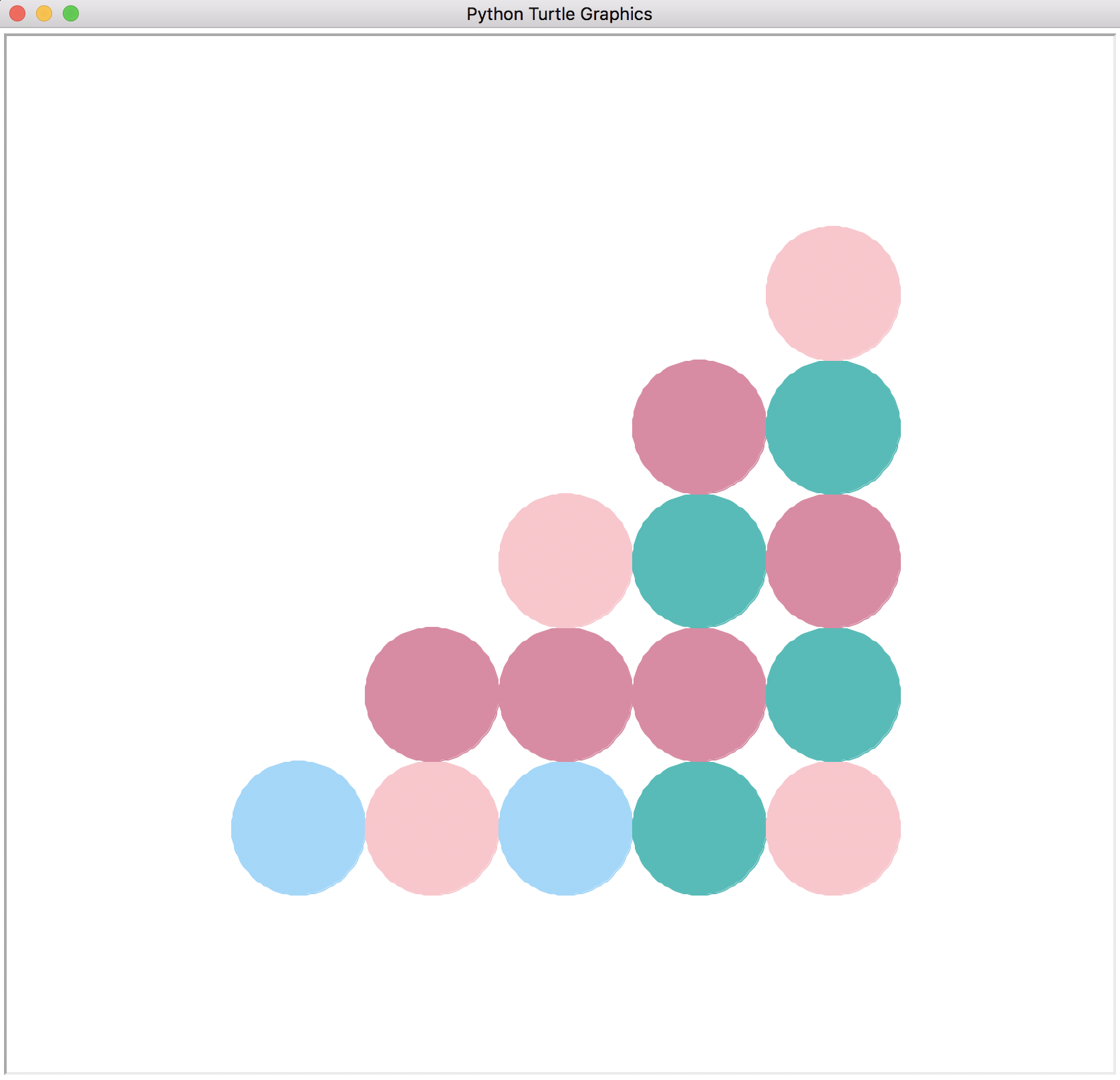Which of the above four patterns is generated by the following code below? Think about it before you run the code below!

In :
for row in range(numRowsAndCols):
for col in range(numRowsAndCols):
if row <= col:
x, y = gridCoordinates(row, col, numRowsAndCols, numRowsAndCols, radius)
color(random.choice(colors))
teleport(x, y)
begin_fill()
end_fill()
In :
reset()
noTrace()
triangleOfCircles(5, 50)
showPicture()
A 1-pensize LightSeaGreen circle centered at (-200, -200) with radius 50
A 1-pensize LightSeaGreen circle centered at (-100, -200) with radius 50
A 1-pensize LightSeaGreen circle centered at (0, -200) with radius 50
A 1-pensize LightSeaGreen circle centered at (100, -200) with radius 50
A 1-pensize LightSeaGreen circle centered at (200, -200) with radius 50
A 1-pensize LightSkyBlue circle centered at (-100, -100) with radius 50
A 1-pensize LightPink circle centered at (0, -100) with radius 50
A 1-pensize LightPink circle centered at (100, -100) with radius 50
A 1-pensize LightSeaGreen circle centered at (200, -100) with radius 50
A 1-pensize LightSeaGreen circle centered at (0, 0) with radius 50
A 1-pensize LightSeaGreen circle centered at (100, 0) with radius 50
A 1-pensize LightPink circle centered at (200, 0) with radius 50
A 1-pensize LightSkyBlue circle centered at (100, 100) with radius 50
A 1-pensize LightPink circle centered at (200, 100) with radius 50
A 1-pensize LightSeaGreen circle centered at (200, 200) with radius 50

Question What (small) changes can we make to the code above to draw the other three patterns?

The code currently produces LowerRightTriangleOfCircles.

• For UpperLeftTriangleOfCircles, change row <= col to row >= col

• For UpperRightTriangleOfCircles, change row <= col to row + col >= numRowsAndCols - 1

• For LowerLeftTriangleOfCircles, change row <= col to row + col <= numRowsAndCols - 1

7. Swapping values¶

The following code will not swap the values correctly:

In :
nums = [3, 2, 1, 4]

# swapping values?
nums = nums
nums = nums
nums
Out:
[2, 2, 1, 4]

What works instead is the use of a so-called temporary variable to hold one of the values temporarily:

In :
nums = [3, 2, 1, 4]

oldNumsSub0 = nums # oldNumSub0 is a temporary variable
nums = nums
nums = oldNumsSub0

nums
Out:
[2, 3, 1, 4]

8. Simultaneous assignment¶

Simulataneous assignment is another name for the notion of tuple assignment introduced near the end of lecture called Iteration1.

Try to guess the result of each cell without running it, then test your guess.

In :
a, b = 0, 1
a + b
Out:
1
In :
a, b, c = 1, 2, 3
print(c, a, b)
3 1 2
In :
a, b = "AB"
print(a, b)
A B
In :
a, b = [10, 20]
print(a + b, a * b)
30 200
In :
a, b = (15, 25)
print(b - a)
10
In :
a, b, c, d = [1, 2, 3, 4]
print(a*b*c*d)
24
In :
a, b = 10
---------------------------------------------------------------------------
TypeError                                 Traceback (most recent call last)
<ipython-input-38-9aefcd17cdb2> in <module>
----> 1 a, b = 10

TypeError: cannot unpack non-iterable int object
In :
a, b = (1, 2, 4)
---------------------------------------------------------------------------
ValueError                                Traceback (most recent call last)
<ipython-input-39-ed6d19501eae> in <module>
----> 1 a, b = (1, 2, 4)

ValueError: too many values to unpack (expected 2)

Swapping variables in one line¶

Using tuple assignment, we can swap values in Python in one statement:

In :
nums = [3, 2, 1, 4]
nums, nums = nums, nums
nums
Out:
[2, 3, 1, 4]
In :
a, b = 0, 1
print(a, b)
a, b = b, a
print(a, b)
0 1
1 0

9. Review: sumBetween with iteration table¶

We have added two print statements in the function, to print the iteration table,
which shows how the three state variables change from one iteration step to the next.

In :
def sumBetween(lo, hi):
"""Returns the sum of the integers from lo to hi
(inclusive). Assume lo and hi are integers.
"""
sumSoFar = 0          # initialize accumulator

# Print initial values of state variables, before entering loop the first time
print('lo:', lo, '| hi:', hi, '| sumSoFar:', sumSoFar)

while lo <= hi:
sumSoFar += lo    # update accumulator
lo += 1
# Print updated values of state variables before start of next iteration of loop
print('lo:', lo, '| hi:', hi, '| sumSoFar:', sumSoFar)

return sumSoFar       # return accumulator
In :
sumBetween(4, 8)
lo: 4 | hi: 8 | sumSoFar: 0
lo: 5 | hi: 8 | sumSoFar: 4
lo: 6 | hi: 8 | sumSoFar: 9
lo: 7 | hi: 8 | sumSoFar: 15
lo: 8 | hi: 8 | sumSoFar: 22
lo: 9 | hi: 8 | sumSoFar: 30
Out:
30

10. Using iteration tables to design loops: replaceVowelSequences¶

When given an iteration problem, it's usually a very bad idea to start by writing Python code. You'll often waste a lot of time implementing and debugging an approach that may be far from correct.

Instead, we recommend that you begin every iteration problem by creating iteration tables for several concrete examples for that problem, and use these to figure out the state variables and iteration rules for the problem. Then you can translate the state variables and iteration rules into Python code.

Here we will illustrate this strategy in the context of a function that appeared on a previous midterm exam.

replaceVowelSequences function specification¶

Define a function replaceVowelSequences that takes a string as its single input, and returns a version of the string in which each sequence of consecutive vowels is replaced by the asterisk character, '*'. The following table shows the output of replaceVowelSequences for some input strings:

Input Output
'section' 's*ct*n'
'conscientious' 'c*nsc*nt*s'
'audacious' '*d*c*s'
'amnesia' '*mn*s*'
'strengths' 'str*ngths'
'wryly' 'wryly'

Some sample iteration tables for replaceVowelSequences¶

In the following iteration tables, explain:

• State Variables: What are the meanings of the state variables resultSoFar and inVowelSequence?

• resultSoFar is the prefix of the final result string determined by the characters processed so far.
• inVowelSequence indicates whether the current char is a vowel. It is helpful, because it can be used in the next row to determine if the previous character was a value.
• Iteration Rules:

• How is resultSoFar in a row determined from (1) char in that row (2) resultSoFar from the previous row and (3) inVowelSequence from the previous row?

• If char is a vowel:
• If the previous inVowelSequence is False, add '*' to the end of resultSoFar
• If the previous inVowelSequence is True, resultSoFar is unchanged
• If char is not a vowel, add char to the end of resultSoFar
• How is inVowelSequence in a row determined from char in that row?

Answer: inVowelSequence is True if char is a vowel and False otherwise.

char resultSoFar inVowelSequence
'' False
's' 's' False
'e' 's*' True
'c' 's*c' False
't' 's*ct' False
'i' 's*ct*' True
'o' 's*ct*' True
'n' 's*ct*n' False
char resultSoFar inVowelSequence
'' False
'a' '*' True
'u' '*' True
'd' '*d' False
'a' '*d*' True
'c' '*d*c' False
'i' '*d*c*' True
'o' '*d*c*' True
'u' '*d*c*' True
's' '*d*c*s' False

Translating the iteration tables into a Python function for replaceVowelSequences¶

Now translate the state variables and iteration rules from above into a Python definition for replaceVowelSequences

In :
def isVowel(char):
return char.lower() in 'aeiou'

def replaceVowelSequences(word):
resultSoFar = ''
inVowelSequence = False
for char in word:
if isVowel(char):
if not inVowelSequence:
resultSoFar += '*'
inVowelSequence = True
else:
resultSoFar += char
inVowelSequence = False
return resultSoFar
In :
def testReplaceVowelSequences(words):
for word in words:
print("replaceVowelSequences('" + word + "') => '" + replaceVowelSequences(word) + "'")

testReplaceVowelSequences('section conscientious audacious amensia strenghts wryly'.split())
replaceVowelSequences('section') => 's*ct*n'
replaceVowelSequences('conscientious') => 'c*nsc*nt*s'
replaceVowelSequences('audacious') => '*d*c*s'
replaceVowelSequences('amensia') => '*m*ns*'
replaceVowelSequences('strenghts') => 'str*nghts'
replaceVowelSequences('wryly') => 'wryly'

An alternative definition of replaceVowelSequences without the inVowelSequence state variable¶

It is possible to determine the value of inVowelSequence by looking at resultSoFar from the previous row. How?

Use this observation to define an alternative definition of replaceVowelSequences without the inVowelSequence state variable

In :
def replaceVowelSequences(word):
resultSoFar = ''
for char in word:
if isVowel(char):
if (resultSoFar == '' # Careful! Must have this test for case
# where word begins with vowel!
or resultSoFar[-1] != '*'):
resultSoFar += '*'
else:
resultSoFar += char
return resultSoFar
In :
testReplaceVowelSequences('section conscientious audacious amensia strenghts wryly'.split())
replaceVowelSequences('section') => 's*ct*n'
replaceVowelSequences('conscientious') => 'c*nsc*nt*s'
replaceVowelSequences('audacious') => '*d*c*s'
replaceVowelSequences('amensia') => '*m*ns*'
replaceVowelSequences('strenghts') => 'str*nghts'
replaceVowelSequences('wryly') => 'wryly'